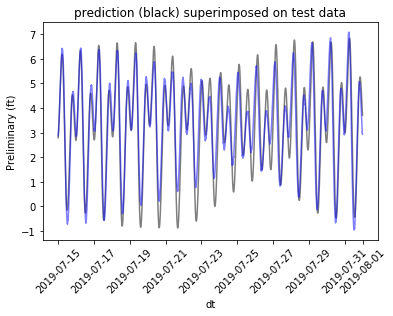# Lord Kelvin, Data Scientist

In 1876 A. Légé & Co., 20 Cross Street, Hatton Gardens, London completed the first “tide calculating machine” for William Thomson (later Lord Kelvin) (ref).

The results were plotted on the paper cylinders, and one literally “turned the crank” to perform the calculations.

The tide calculating machine embodied ideas of Sir Isaac Newton, and Pierre-Simon Laplace (ref), and could predict tide driven water levels by the means of wheels and gears.

The question is: can modern data science tools quickly forecast tides to similar accuracy?

In one sense the answer is “yes”. The common data science toolkit contains complete modules for specialized tasks such as tide prediction. In R one such package is TideHarmonics. Python has a similar package named pytides.

These packages use the harmonic terms developed by George Darwin and A T Doodson (ref) to give us a set of frequencies or tidal constituent periods we can use to model tides using historic measurements. The models tend to have an accuracy and lifetime typically unheard of by modern data scientists. For example below is an R-produced July 2019 extrapolation for NOAA’s 9414290 San Francisco tide predictions plotted on top of later measured water levels.The predictions are so close to later observed actuals we have a hard time telling the two curves apart. The R-squared between predictions and actuals is around 0.99, as seen in the following scatter plot showing actual as a function of predicted.So that is how well 19th century scientists and oceanographers expected their predictions to be. The equivalent R-code is given here.

But what happens if we do not have access to the tide machine (or at least the ability to copy the Doodson frequencies off its rotors!)?

We could try to infer the tidal frequencies from data using the Fast Fourier Transform (FFT) and then fit a combination of these frequencies to our historic data using Elastic Net Regularized Linear Regression. An example of trying to do this (this time using Python) can be found here. The results look like this:This has an out of sample R-squared of 0.81. If we hadn’t seen the 19th century results, we might be fooled into thinking this is good (and it is certainly better than one gets through a superficial application of ARIMA methods).

What is going wrong in the above extrapolation is: we didn’t learn the true frequencies or periods of the forces driving the tidal effects. For example Doodson’s Lunar monthly term Mm has a period of 661.3111655 hours per cycle. The data we have is sampled regularly at 6-minute intervals. So straightforward signal analysis methods are only going to discover approximate terms with with periods evenly divisible by 6 minutes, or even tenths of hours. The correct modeling frequencies are not in the concept set of the basic modeling idea. The model can memorize short intervals of training data, and extrapolate for short periods; but the model is incorrect and falls out of sync on longer prediction intervals. Of course, given we can measure what is going wrong on long data sets, we can work out ways to critique and estimate proposed frequencies/harmonics.

Let’s try modeling by hand again. This time instead of using FFT methods to guess the important frequencies we steal them from the vetted tidal models. We could get these frequencies from the theory of tides article, NOAA, or (as we did) dumping TidalHarmonics’s table.

The idea is: the tidal prediction turns out to be a sum of terms of the form `Ai cos(ai t + αi)` (ref). The `ai` are the tidal frequencies or harmonics, and the other terms are easily found by regression (using the equality `cos(ai t + αi) = cos(αi) cos(ai t) + sin(αi) sin(ai t)`; meaning: if we add `cos(ai t)` and `sin(ai t)` as two variables, that is enough to exactly match any function of the form `Ai cos(ai t + αi)`).

Using our Elastic Net regression with the imported frequency table gives us a Python result indistinguishable from using a complete tide package (link).

So in conclusion: modern data science methods can be competitive with 19th century tide calculation machines, but in this example only after we incorporated some of the actual original science (celestial mechanics, harmonic analysis, and tidal theory). The 19th century scientists were working at an accuracy and scale of predictions (projecting whole years of tides at once) that general “close is good enough” tooling has a hard time reproducing their results.

Edit August 8, 2019: Fred Viole shared a great “data only” solution using his NNS package. Please check it and the NNS package out.### jmount

Data Scientist and trainer at Win Vector LLC. One of the authors of Practical Data Science with R.